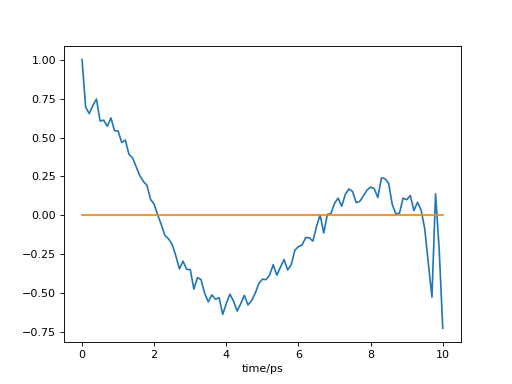# Utilities¶

## Module: utilities¶

`pytim.utilities.``correlate`(a1, a2=None, _normalize=True)

correlate data series using numpy fft. The function calculates correlation or cross-correlation.

Parameters: a1 (ndarray) – first data set to correlate a2 (ndarray) – (optional) second data set, to compute the cross-correlation
Example: time autocorrelation of the number of atoms in the outermost
layer
```>>> import MDAnalysis as mda
>>> import pytim
>>> import numpy as np
>>> from pytim.datafiles import *
>>>
>>> u = mda.Universe(WATER_GRO,WATER_XTC)
>>> inter = pytim.ITIM(u)
>>>
>>> size=[]
>>> time=[]
>>> # sample the size of the first layer on the upper
>>> # side
>>> for ts in u.trajectory[:50]:
...     time.append(ts.time)
...     size.append(len(inter.layers[0,0]))
>>>
>>> # we need to subtract the average value
>>> np.set_printoptions(precision=3)
>>> corr = pytim.utilities.correlate(size-np.mean(size))
>>> corr = corr/corr # normalize to 1
>>> print (corr)
[ 1.     0.142  0.104  0.147  0.371  0.099  0.165  0.095  0.338  0.219
-0.021  0.087  0.245 -0.01  -0.193  0.103  0.029 -0.009 -0.11   0.012
-0.133  0.056 -0.283 -0.276  0.035 -0.012 -0.211 -0.429 -0.132 -0.263
0.072 -0.7   -0.236  0.136 -0.243 -0.878 -0.13  -0.329 -0.386 -0.652
-0.267 -0.188 -0.226 -0.79  -0.284 -0.02  -1.512 -1.316 -0.188  7.551]
```
```>>> np.set_printoptions()
```

This will produce (sampling the whole trajectory), the following:`pytim.utilities.``guess_normal`(universe, group)

Guess the normal of a liquid slab

`pytim.utilities.``lap`(show=False)

Timer function

Parameters: show (bool) – (optional) print timer information to stderr